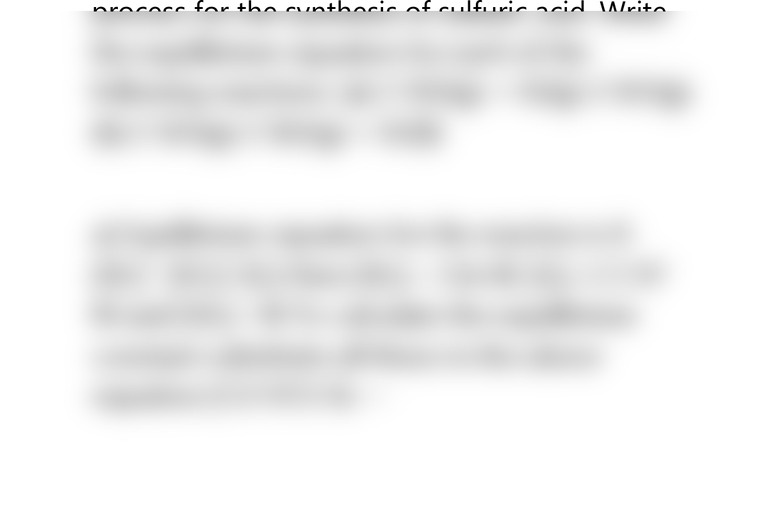# CAD-8104 Lecture Notes - Lecture 17: Sulfur Dioxide, Equilibrium Constant

6 views2 pages
School
Department
Course
ProfessorCHEM 2426 HOMEWORK SOLUTIONS
The following equilibrium concentrations were
measured at 800 K: [30,] = 3.0 X 10-3M; [0,] =
3.5 X 10-3M;  = 5.0 X 10-2M. Calculate the
equilibrium constant at 800 K for each of the
reactions in Problem.
Problem
The oxidation of sulfur dioxide to give sulfur
trioxde is an important step In the industrial
process for the synthesis of sulfuric acid. Write
the equilibrium equation for each of the
following reactions: (a) 2 S02(g) + 02(g) 2 S03(g)
(b) 2 S03(g) 2 S02(g) + 02(8)
a) Equilibrium equation for the reaction is K
[S0,]' [SO,]' [0,] Here [S0,]= 3.0x M; [0,]=3.5.10'
M and [S0,]= M To calculate the equilibrium
constant substitute all these in the above
equation [5.0.1012 Kc
Unlock document

This preview shows half of the first page of the document.
Unlock all 2 pages and 3 million more documents.